Question

# How does the impedance of an electrode change with its surface area?  (increase / decrease) How does...

1. How does the impedance of an electrode change with its surface area?  (increase / decrease)
2. How does the parallel capacitance (double-charge layer capacitance) of an electrode change by its surface area?   (increase / decrease)
3. Draw the electrical equivalence of an electrode/electrolyte interface using 4 passive electrical components.

1. The impedance is inversely proportional to the surface area so as the surface area of electrode increases its impedance decreases.

2.the parallel capacitance of an electrode is directly proportional to the surface area of electrode ao as the surface area increases the parallel capacitance also increases.

Pleaee post the last question seperately.. Thanx

#### Earn Coins

Coins can be redeemed for fabulous gifts.

Similar Homework Help Questions
• ### 9. How can the lamprey increase the available surface area of its intestines? What does this...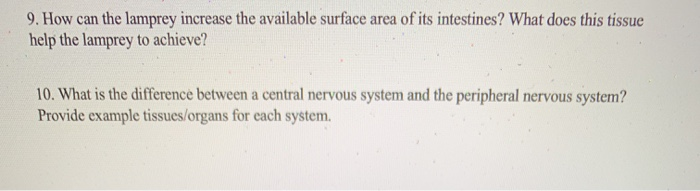9. How can the lamprey increase the available surface area of its intestines? What does this tissue help the lamprey to achieve? 10. What is the difference between a central nervous system and the peripheral nervous system? Provide example tissues/organs for each system.

• ### (1) As shown in the Figure below, a parallel-plate capacitor of plate area A is filled with two laye ers of dielectrics, di and de thick, with permittivities s and s2, respectively. Ignoring the r...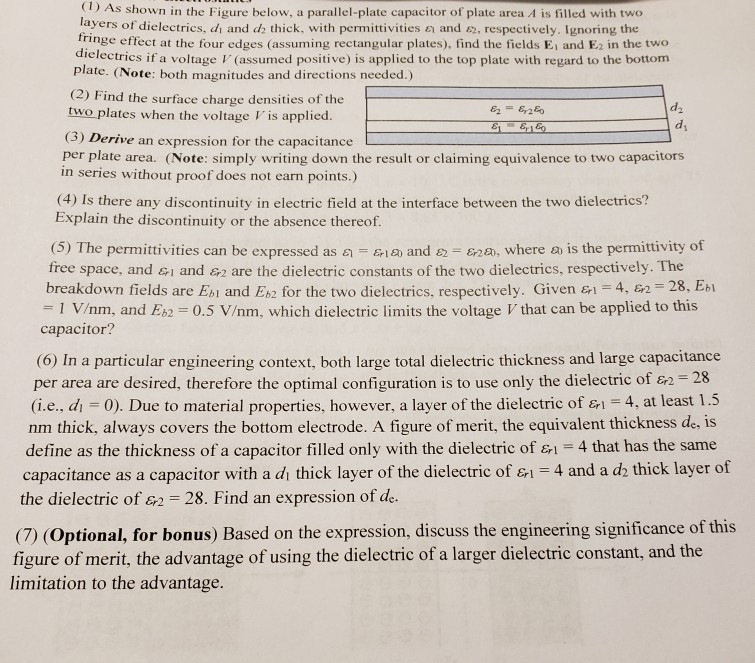(1) As shown in the Figure below, a parallel-plate capacitor of plate area A is filled with two laye ers of dielectrics, di and de thick, with permittivities s and s2, respectively. Ignoring the ringe effect at the four edges (assuming rectangular plates), find the fields Ei and E2 in the t dielectric ctrics if a voltage V(assumed positive) is applied to the top plate with regard to the bottom plate. (Note: both magnitudes and directions needed.) (2) Find the...

• ### Problem 1. Electrostatics (1) As shown in the Figure below, a parallel-plate capacitor of plate area A is filled with two layers of dielectrics, ch and d2 thick, with permittivities fringe effect...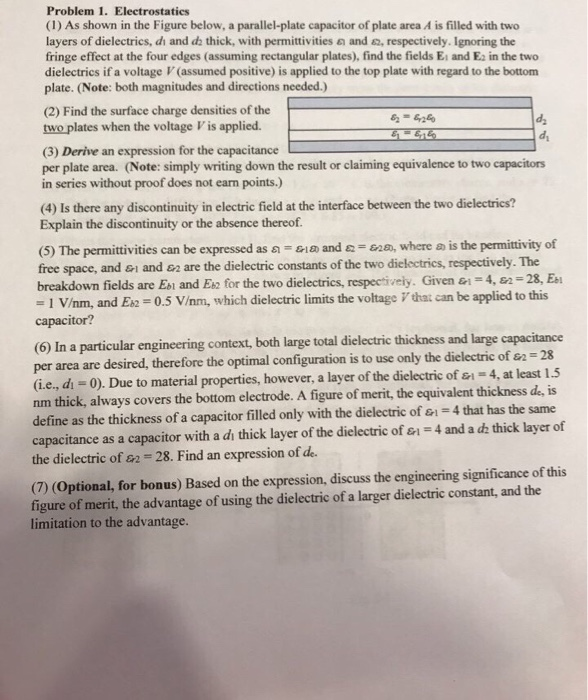Problem 1. Electrostatics (1) As shown in the Figure below, a parallel-plate capacitor of plate area A is filled with two layers of dielectrics, ch and d2 thick, with permittivities fringe effect at the four edges (assuming rectangular plates), find the fields Ei and Es in the two dielectrics if a voltage V (assumed positive) is applied to the top plate with regard to the bottom plate. (Note: both magnitudes and directions needed.) and a, respectively. Ignoring the (2) Find...

• ### Suppose you have a following reaction: How does the reaction rate change increase, decrease, does not...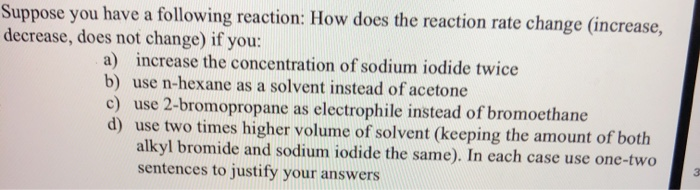Suppose you have a following reaction: How does the reaction rate change increase, decrease, does not change) if you: a) increase the concentration of sodium iodide twice b) use n-hexane as a solvent instead of acetone c) use 2-bromopropane as electrophile instead of bromoethane d) use two times higher volume of solvent (keeping the amount of both alkyl bromide and sodium iodide the same). In each case use one-two sentences to justify your answers CH3CH3Br + Nal acetone → CH2CH3...

• ### b. Does the resistance of the electric light bulb increase, decrease or remain constant as the...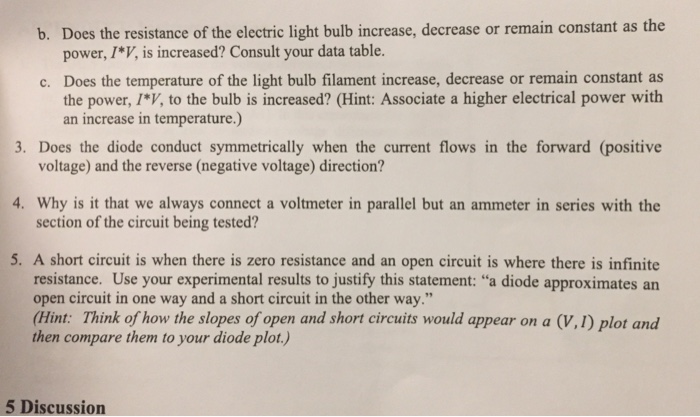b. Does the resistance of the electric light bulb increase, decrease or remain constant as the power, I*V, is increased? Consult your data table. Does the temperature of the light bulb filament increase, decrease or remain constant as c. the power, IV, to the bulb is increased? (Hint: Associate a higher electrical power with 3. Does the diode conduct symmetrically when the current flows in the forward (positive 4. Why is it that we always connect a voltmeter in parallel...

• ### A model of a red blood cell portrays the cell as a spherical capacitor, a positively...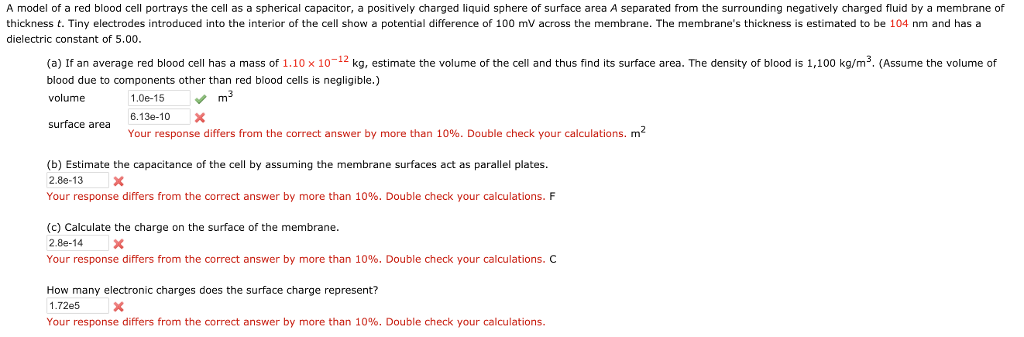A model of a red blood cell portrays the cell as a spherical capacitor, a positively charged liquid sphere of surface area A separated from the surrounding negatively charged fluid by a membrane of thickness t. Tiny electrodes introduced into the interior of the cell show a potential difference of 100 mV across the membrane. The membrane's thickness is estimated to be 104 nm and has a dielectric constant of 5.00 (a) If an average red blood cell has a...

• ### Does anybody know how to create a score of increase and decrease using javascript ? I...

Does anybody know how to create a score of increase and decrease using javascript ? I will like to create a score system where If the user decides to not guess any number and just get what ever number that is given to them then there points is just what ever number comes up. For example: if the user just clicks on the no random button they just get what ever number comes up If the user wants to guess...

• ### please answer 1-6 and 8 DISCUSSION 1. Does the solubility of borax increase or decrease when...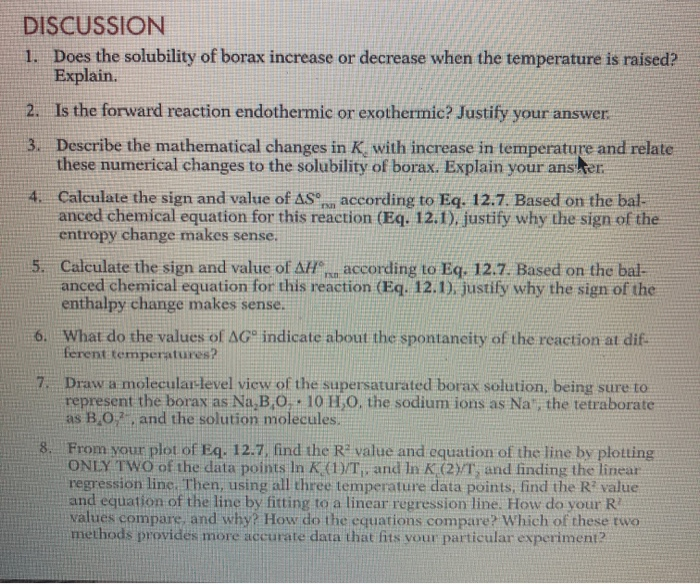please answer 1-6 and 8 DISCUSSION 1. Does the solubility of borax increase or decrease when the temperature is raised? Explain. 2. Is the forward reaction endothermic or exothermic? Justify your answer 3. Describe the mathematical changes in K, with increase in temperature and relate these numerical changes to the solubility of borax. Explain your anster 4. Calculate the sign and value of AS according to Eq. 12.7. Based on the bal- anced chemical equation for this reaction (Eq. 12.1),...

• ### what is b plz . Consider a disconnected parallel plate capacitor with area Ao and width --4, 3do. There is a magnitude of charge density g, on each plate. For this problem you may assume the plates ar...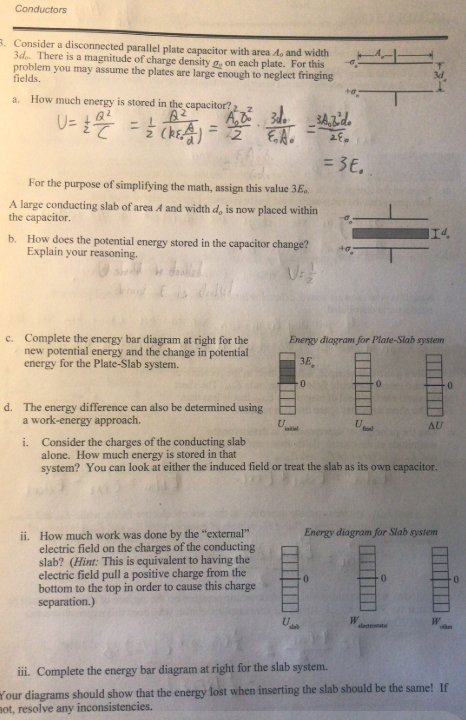what is b plz . Consider a disconnected parallel plate capacitor with area Ao and width --4, 3do. There is a magnitude of charge density g, on each plate. For this problem you may assume the plates are large enough to neglect fringing fields. 3d How much energy is stored in the capacitor?2 z a. For the purpose of simplifying the math, assign this value 3E. A large conducting slab of area A and width do is now placed within...